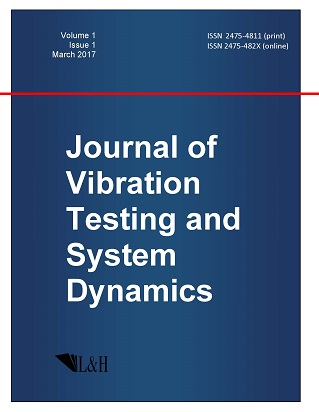ISSN: 2475-4811 (print)
ISSN: 2475-482X (online)
Journal of Vibration Testing and System Dynamics

C. Steve Suh (editor), Pawel Olejnik (editor),

Xianguo Tuo (editor)

Pawel Olejnik (editor)

Lodz University of Technology, Poland

Email: pawel.olejnik@p.lodz.pl

C. Steve Suh (editor)

Texas A&M University, USA

Email: ssuh@tamu.edu

Xiangguo Tuo (editor)

Sichuan University of Science and Engineering, China

Email: tuoxianguo@suse.edu.cn

Bifurcation Trees of (1:2)-Asymmetric Periodic Motions with Corresponding Infinite Homoclinic Orbits in the Lorenz System

Journal of Vibration Testing and System Dynamics 5(4) (2021) 373--406 | DOI:10.5890/JVTSD.2021.12.005

Siyu Guo, Albert C.J. Luo

Department of Mechanical and Industrial Engineering, Southern Illinois University Edwardsville, Edwardsville, IL 62026-1805, USA

Abstract

In this paper, bifurcation dynamics and infinite homoclinic orbits of (1:2)- asymmetric periodic motions in the Lorenz systems are studied through the discrete mapping method. The infinite homoclinic orbits pertaining to the unstable periodic motions on the bifurcation trees of (1:2)-asymmetric periodic motions to chaos are determined. The stability and bifurcations of periodic motions are determined through the eigenvalue analysis. A bifurcation tree of (1:2) asymmetric periodic motions, varying with the Rayleigh number, is presented by discrete nodes, and the corresponding harmonic frequency-amplitude characteristics of periodic motions on the bifurcation tree are discussed through finite Fourier series analysis. A sequence of period-doubling bifurcations is observed on the bifurcation tree of the (1:2)- asymmetric periodic motion to chaos. The scenario of (1:2)- asymmetric period-1, period-2, period-4 motions to chaos scenario is illustrated. Homoclinic orbits are as appearance or vanishing of unstable periodic motions on the bifurcation tree. Illustrations of periodic motions and homoclinic orbits are completed for intuitive demonstrations of the corresponding topological structures. This study extends the initial study of the bifurcation tree from the (1:1)-symmetric periodic motions to asymmetric periodic motions then to chaos in the Lorenz system, and the corresponding infinite homoclinic orbits induced by all unstable periodic motions can be determined. Such results can enrich the understanding of bifurcation dynamics of asymmetric periodic motions to chaos in the Lorenz system.

References

1.   Lorenz, E.N. (1963), Deterministic nonperiodic flow, Journal of the atmospheric sciences, 20(2), 130-141.
2.   Curry, J.H. (1978), A generalized Lorenz system, Communications in Mathematical Physics, 60(3), 193-204.
3.   Tucker, W. (1999), The Lorenz attractor exists, Comptes Rendus de lAcad\{emie des Sciences-Series I-Mathematics}, 328(12), 1197-1202.
4.   Shilnikov, L.P. (1967), On a Poincar\{e}--Birkhoff problem, Matematicheskii Sbornik, 116(3), 378-397.
5.   Hastings, S.P. and Troy, W.C. (1994), A proof that the Lorenz equations have a homoclinic orbit, Journal of Differential Equations, 113(1), 166-188.
6.   Gavrilov, N.K. and Shilnikov, L.P. (1973), On three-dimensional dynamical systems close to systems with a structurally unstable homoclinic curve. II, Mat. Sb. ($N.S$.), 90, 139-156
7.   Afraimovich, V.S., Bykov, V.V., and Shilnikov, L.P. (1977), Origin and structure of the Lorenz attractor, Doklady Akademii nauk SSSR, 234, 336-339.
8.   Rand, D. (1978), The topological classification of Lorenz attractors, Math. Proc. Cambridge Philos. Soc, 83(3), pp.451-460.
9.   Guckenheimer, J. and Williams, R.F. (1979), Structural stability of Lorenz attractors, Publications Math\{ematiques de lInstitut des Hautes \{E}tudes Scientifiques}, 50(1), 59-72.
10.   Williams, R.F. (1979), The structure of Lorenz attractors, Publications Mathematiques de lIHES, 50, 73-99.
11.   Robbins, K.A. (1979), Periodic solutions and bifurcation structure at high R in the Lorenz model, SIAM Journal on Applied Mathematics, 36(3), 457-472.
12.   Lyubimov, D.V. and Zaks, M.A. (1983), Two mechanisms of the transition to chaos in finite-dimensional models of convection, Physica D: Nonlinear Phenomena, 9(1-2), 52-64.
13.   Fr{\o}yland, J. and Alfsen, K.H. (1984), Lyapunov-exponent spectra for the Lorenz model, Physical Review A, 29(5), 2928-2931.
14.   Shimizu, T. and Morioka, N. (1978), Chaos and limit cycles in the Lorenz model, Physics Letters A, 66(3), 182-184.
15.   Kaplan, J.L. and Yorke, J.A. (1979) Preturbulence: a regime observed in a fluid flow model of Lorenz, Communications in Mathematical Physics, 67(2), 93-108.
16.   Jackson, E.A. (1985), The Lorenz system: I. The global structure of its stable manifolds, Physica Scripta, 32(5), 469-475.
17.   Jackson, E.A. (1985), The Lorenz system: II. The homoclinic convolution of the stable manifolds, Physica Scripta, 32(5), 476-481.
18.   Yorke, J.A. and Yorke, E.D. (1979), Metastable chaos: The transition to sustained chaotic behavior in the Lorenz model, Journal of Statistical Physics, 21(3), 263-277.
19.   Sparrow, C. (1983), An introduction to the Lorenz equations, IEEE Transactions on Circuits and Systems, 30(8), 533-542.
20.   Vakakis, A.F. and Azeez, M.F.A. (1998), Analytic approximation of the homoclinic orbits of the Lorenz system at $\sigma =10$, $b = 8/3$ and $\rho = 13.926$... Nonlinear Dynamics, 15(3), 245-257.
21.   Saha, P., Saha, D.C., Ray, A., and Chowdhury, A.R. (2018), On some properties of memristive Lorenz equation--theory and experiment, Journal of Applied Nonlinear Dynamics, 7(4), pp.413-423.
22.   Liu, X. and Hong, L. (2015), The adaptive synchronization of the stochastic fractional-order complex Lorenz system, Journal of Applied Nonlinear Dynamics, 4(3), 267-279.
23.   Hassan, S.S. (2016), Computational complex dynamics of the discrete Lorenz system, Journal of Applied Nonlinear Dynamics, 8(3), 345-366.
24.   Luo, A.C.J. (2015), Discretization and implicit mapping dynamics, Springer Berlin Heidelberg.
25.   Luo, A.C.J. (2015), Periodic flows to chaos based on discrete implicit mappings of continuous nonlinear systems, International Journal of Bifurcation and Chaos, 25(03), 1550044.
26.   Guo, Y. and Luo, A.C.J, (2015), On complex periodic motions and bifurcations in a periodically forced, damped, hardening Duffing oscillator. Chaos, Solitons {$\&$ Fractals}, 81, 378-399.
27.   Luo, A.C.J. and Xing, S. (2016), Analytical predictions of period-1 motions to chaos in a periodically driven quadratic nonlinear oscillator with a time-delay, Mathematical Modelling of Natural Phenomena, 11(2), 75-88.
28.   Xu, Y., Chen, Z., and Luo, A.C.J. (2020), An independent period-3 motion to chaos in a nonlinear flexible rotor system, International Journal of Dynamics and Control, 8, 337-351.
29.   Guo, C. and Luo, A.C.J. (2021), Periodic motions on bifurcation trees in an inverted pendulum with a periodically moving base, International Journal of Dynamics and Control, 9, 410-423.
30.   Guo, S. and Luo, A.C.J. (2021), On infinite homoclinic orbits induced by unstable periodic orbits in the Lorenz system, Chaos, 31, 043106 (14 pages).
31.   Luo, A.C.J. (2012), Continuous Dynamical Systems, HEP/L&H scientific, Beijing/Glen Carbon.